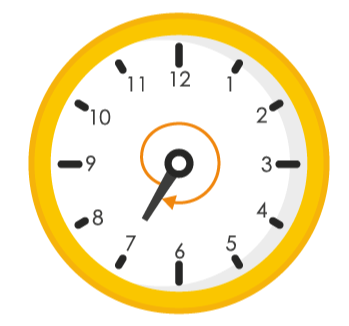## Access NCERT Solutions for Class 6 Chapter 5: Understanding Elementary Shapes Exercise 5.2

### Access NCERT Solutions for Class 6 Chapter 5: Understanding Elementary Shapes Exercise 5.2

1. What fraction of a clockwise revolution does the hour hand of a clock turn through, when it goes from

(a) 3 to 9

(b) 4 to 7

(c) 7 to 10

(d) 12 to 9

(e) 1 to 10

(f) 6 to 3

Solutions:

We know that in one complete clockwise revolution, hour hand will rotate by 3600

(a) When hour hand goes from 3 to 9 clockwise, it will rotate by 2 right angles or 1800

∴ Fraction = 1800 / 3600

= 1 / 2(b) When hour hand goes from 4 to 7 clockwise, it will rotate by 1 right angle or 900

∴ Fraction = 900 / 3600

= 1 / 4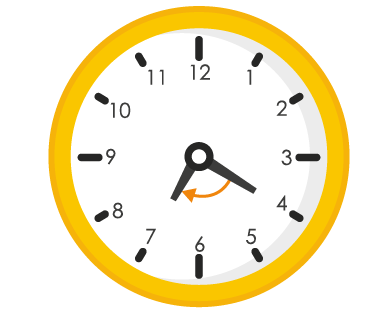(c) When hour hand goes from 7 to 10 clockwise, it will rotate by 1 right angle or 900

∴ Fraction = 900 / 3600

= 1 / 4(d) When hour hand goes from 12 to 9 clockwise, it will rotate by 3 right angles or 2700

∴ Fraction = 2700 / 3600

= 3 / 4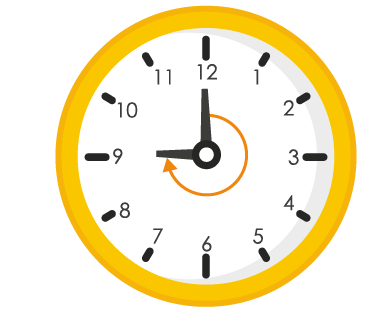(e) When hour hand of a clock goes from 1 to 10 clockwise, it will rotate by 3 right angles or 2700

∴ Fraction = 2700 / 3600

= 3 / 4(f) When hour hand goes from 6 to 3 clockwise, it will rotate by 3 right angles or 2700

∴ Fraction = 2700 / 3600

= 3 / 4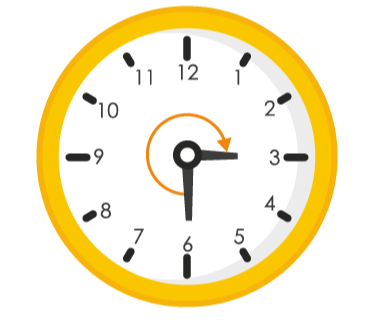2. Where will the hand of a clock stop if it

(a) starts at 12 and makes 1 / 2 of a revolution, clockwise?

(b) starts at 2 and makes 1 / 2 of a revolution, clockwise?

(c) starts at 5 and makes 1 / 4 of a revolution, clockwise?

(d) starts at 5 and makes 3 / 4 of a revolution, clockwise?

Solutions:

We know that one complete clockwise revolution, hour hand will rotate by 3600

(a) When hour hand of a clock starts at 12 and makes 1 / 2 revolution clockwise, it will rotate by 1800.

Hence, the hour hand of a clock will stop at 6.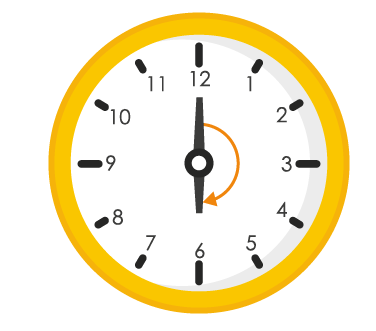(b) When hour hand of a clock starts at 2 and makes 1 / 2 revolution clockwise, it will rotate by 1800

Hence, the hour hand of a clock will stop at 8.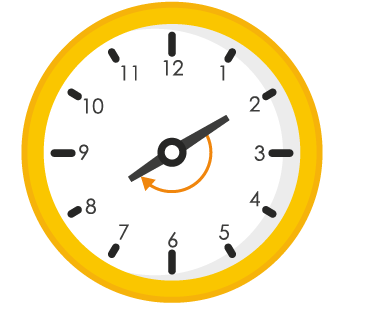(c) When hour hand of a clock starts at 5 and makes 1 / 4 revolution clockwise, it will rotate by 900

Hence, hour hand of a clock will stop at 8.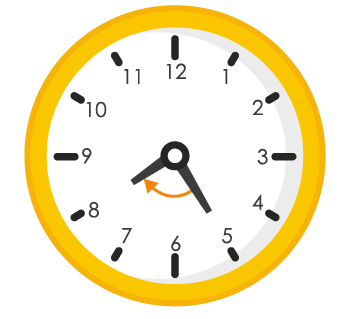(d) When hour hand of a clock starts at 5 and makes 3 / 4 revolution clockwise, it will rotate by 2700

Hence, hour hand of a clock will stop at 2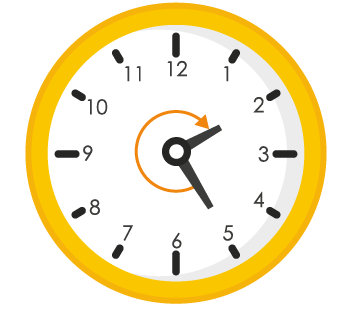3. Which direction will you face if you start facing

(a) east and make 1 / 2 of a revolution clockwise?

(b) east and make 1 ½ of a revolution clockwise?

(c) west and make 3 / 4 of a revolution anti – clockwise?

(d) south and make one full revolution?

(should we specify clockwise or anti – clockwise for this last question? Why not?)

Solutions:

Revolving one complete round in clockwise or in anti – clockwise direction we will revolve by 3600 and two adjacent directions are at 900 or 1 / 4 of a complete revolution away from each other.

(a) If we start facing towards East and make 1 / 2 of a revolution clockwise, we will face towards West direction.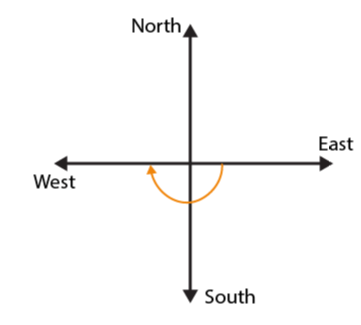(b) If we start facing towards East and make 1 ½ of a revolution clockwise, we will face towards West direction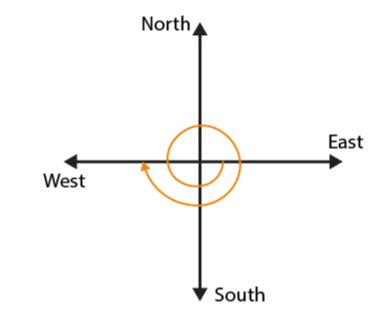(c) If we start facing towards West and make 3 / 4 of a revolution anti – clockwise, we will face towards North direction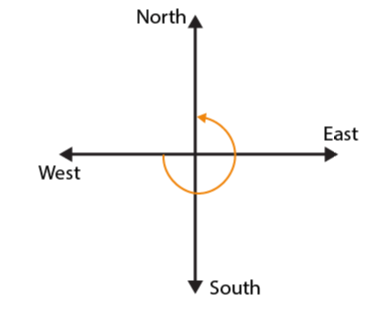(d) If we start facing South and make one full revolution, again we will face the South direction.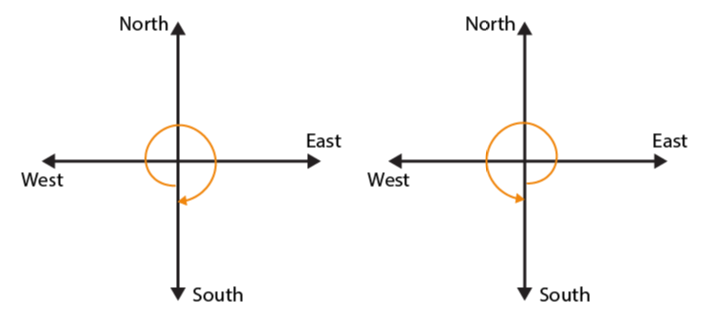In case of revolving 1 complete revolution, either clockwise or anti-clockwise we will be back at the original position.

4. What part of a revolution have you turned through if you stand facing

(a) east and turn clockwise to face north?

(b) south and turn clockwise to face east

(c) west and turn clockwise to face east?

Solutions:

By revolving one complete revolution either in clockwise or in anti-clockwise direction, we will revolve by 3600 and two adjacent directions are at 900 or 1 / 4 of a complete revolution away from each other

(a) If we start facing towards East and turn clockwise to face North, we have to make 3 / 4 of a revolution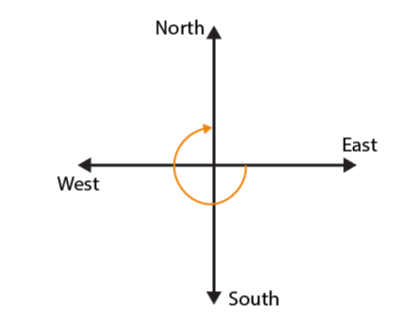(b) If we start facing towards South and turn clockwise to face East, we have to make 3 / 4 of a revolution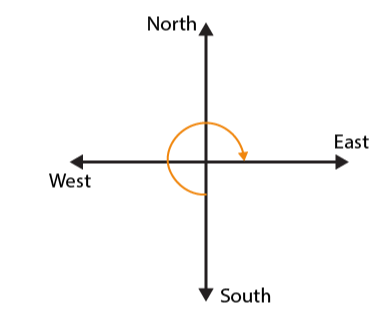(c) If we start facing towards West and turn clockwise to face East, we have to make 1 / 2 of a revolution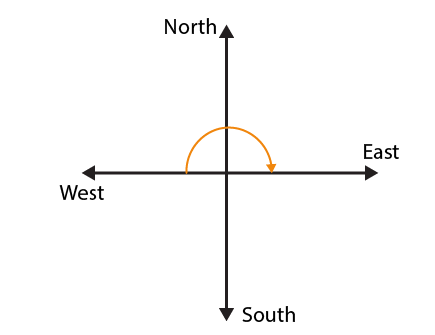5. Find the number of right angles turned through by the hour hand of a clock when it goes from

(a) 3 to 6

(b) 2 to 8

(c) 5 to 11

(d) 10 to 1

(e) 12 to 9

(f) 12 to 6

Solutions:

The hour hand of a clock revolves by 3600 or it covers 4 right angles in one complete revolution

(a) If hour hand of a clock goes from 3 to 6, it revolves by 900 or 1 right angle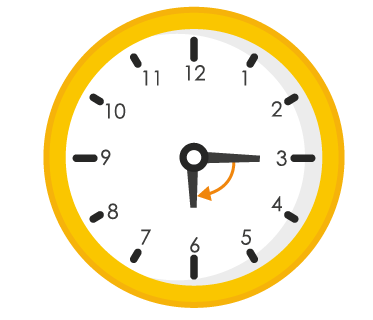(b) If hour hand of a clock goes from 2 to 8, it revolves by 1800 or 2 right angles(c) If hour hand of a clock goes from 5 to 11, it revolves by 1800 or 2 right angles(d) If hour hand of a clock goes from 10 to 1, it revolves by 900 or 1 right angle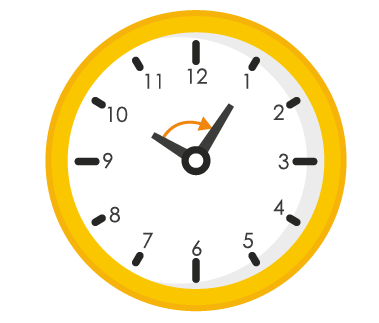(e) If hour hand of a clock goes from 12 to 9, it revolves by 2700 or 3 right angles(f) If hour hand of a clock goes from 12 to 6, it revolves by 1800 or 2 right angles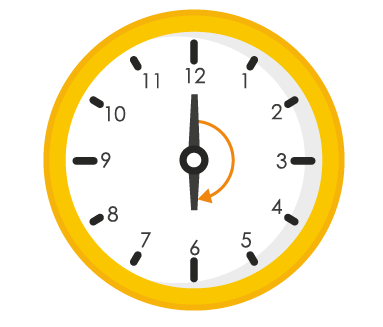6. How many right angles do you make if you start facing

(a) south and turn clockwise to west?

(b) north and turn anti – clockwise to east?

(c) west and turn to west?

(d) south and turn to north?

Solutions:

By revolving one complete round in either clockwise or anti-clockwise direction, we will revolve by 3600 and two adjacent directions are at 900 away from each other.

(a) If we start facing towards South and turn clockwise to West, we have to make one right angle(b) If we start facing towards North and turn anti-clockwise to East, we have to make 3 right angles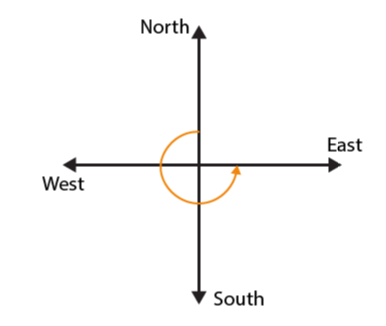(c) If we start facing towards West and turn to West, we have to make one complete round or 4 right angles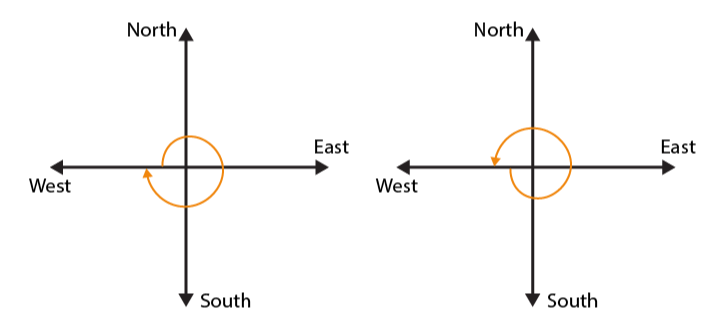(d) If we start facing towards South and turn to North, we have to make 2 right angles7. Where will the hour hand of a clock stop if it starts

(a) from 6 and turns through 1 right angle?

(b) from 8 and turns through 2 right angles?

(c) from 10 and turns through 3 right angles?

(d) from 7 and turns through 2 straight angles?

Solutions:

We know that in 1 complete revolution in either clockwise or anticlockwise direction, hour hand of a clock will rotate by 3600 or 4 right angles

(a) If hour hand of a clock starts from 6 and turns through 1 right angle, it will stop at 9(b) If hour hand of a clock starts from 8 and turns through 2 right angles, it will stop at 2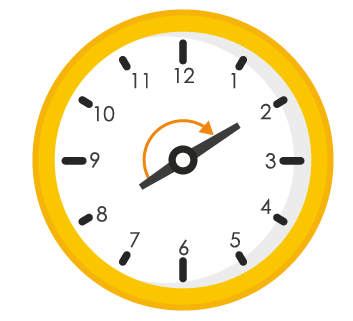(c) If hour hand of a clock starts from 10 and turns through 3 right angles, it will stop at 7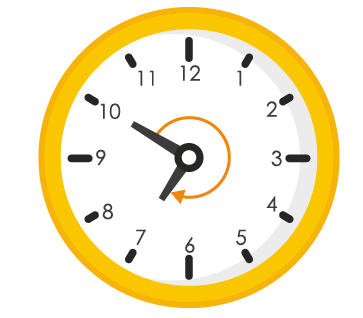(d) If hour hand of a clock starts from 7 and turns through 2 straight angles, it will stop at 7